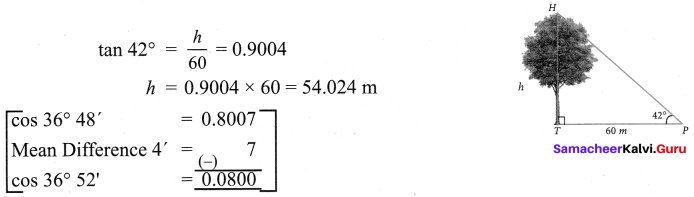Samacheer Kalvi 9th Maths Solutions Chapter 6 Trigonometry Ex 6.4

Tamilnadu Samacheer Kalvi 9th Maths Solutions Chapter 6 Trigonometry Ex 6.4

Question 1.
Find the value of the following :
(i) sin 49°
(ii) cos 74° 39′
(iii) tan 54° 26′
(iv) sin 21° 21′
(v) cos 33° 53′
(vi) tan 70° 17′
Solution: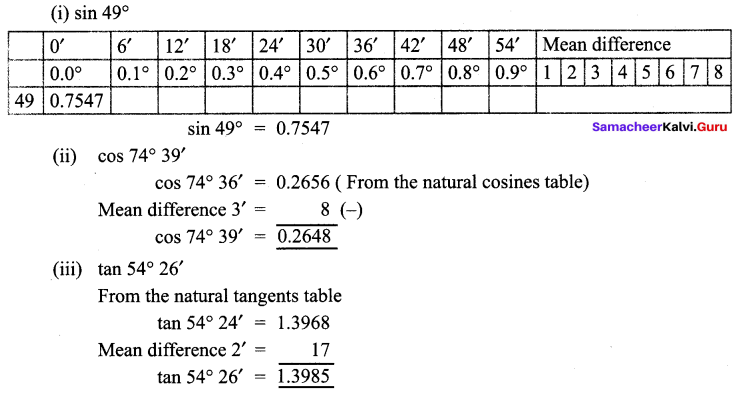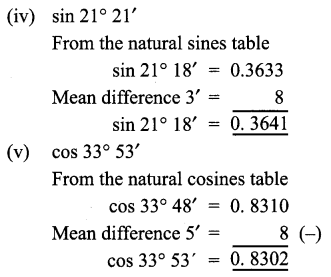Question 2.
Find the value of θ if
(i) sin θ = 0.9975
(ii) cos θ = 0.6763
(iii) tan θ = 0.0720
(iv) cos θ = 0.0410
(v) tan θ = 7.5958
Solution:
(i) From the natural sines table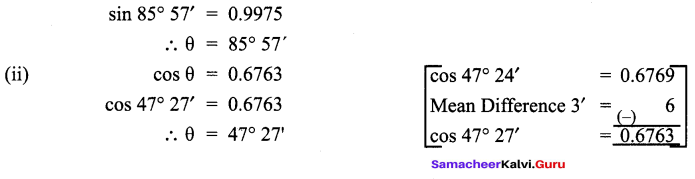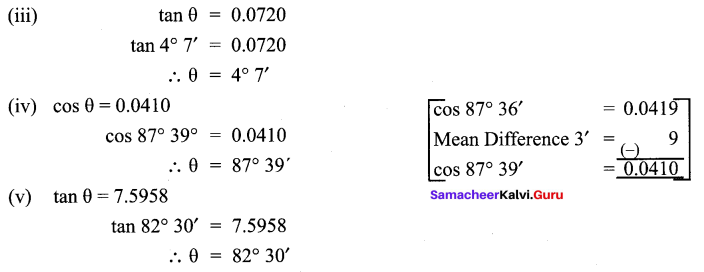Question 3.
Find the value of the following :
(i) sin 65° 39′ + cos 24° 57′
(ii) tan 70° 58′ + cos 15° 26′ – sin 84° 59′
Solution:
(i) = 0.9111 + 0.9066 + 0.1793 = 1.9970
(ii) = 2.8982 + 0.9639 – 0.9962 = 3.8621 – 0.9962 = 2.8659

Question 4.
Find the area of a right triangle whose hypotenuse is 10cm and one of the acute angle is 24° 24′
Solution: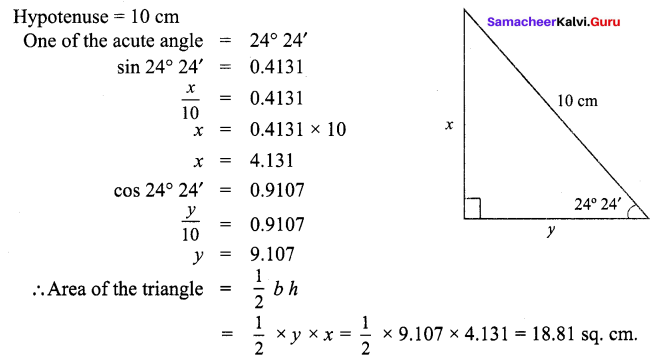Question 5.
Find the angle made by a ladder of length 5m with the ground, if one of its end is
4m away from the wall and the other end is on the wall.
Solution: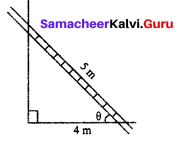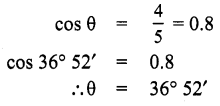Question 6.
In the given figure, HT shows the height of a tree standing vertically. From a point P, the angle of elevation of the top of the tree (that is ∠P) measures 42° and the distance to the tree is 60 metres. Find the height of the tree.
Solution: